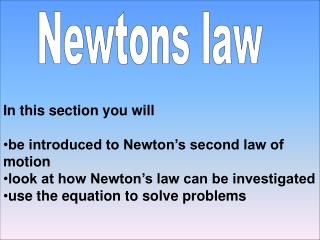Download PresentationNewtons law

# Newtons law

Télécharger la présentation## Newtons law

- - - - - - - - - - - - - - - - - - - - - - - - - - - E N D - - - - - - - - - - - - - - - - - - - - - - - - - - -
##### Presentation Transcript

1. Newtons law • In this section you will • be introduced to Newton’s second law of • motion • look at how Newton’s law can be investigated • use the equation to solve problems

2. Newtons law Isaac Newton said that:- An object will remain stationary or move at a unless a resultant acts on it.

3. Newtons law Isaac Newton said that:- An object will remain stationary or move at a constant velocity unless a resultant force acts on it.

4. EXPERIMENT 1 The air track removes the force of friction. The only force will be exerted by the weights. You will see how increasing the force on the glider affects it’s acceleration

5. Investigating Newton’s law GLIDER Bench Bench 2

6. Investigating Newton’s law GLIDER Bench Bench 2

7. Investigating Newton’s law GLIDER Bench Bench 2

8. Investigating Newton’s law GLIDER Bench Bench 2

9. Force GLIDER Bench 10g Mass holder Bench Weight 2 Fill in the missing numbers To show you understand the pattern (trend) Click to check your answers

10. Force GLIDER Bench 10g Mass holder Bench Weight 2

11. EXPERIMENT 2 The air track removes the force of friction. The only force will be exerted by the weights. You will see how increasing the mass of the glider affects it’s acceleration

12. Investigating Newton’s law GLIDER Bench Bench 2

13. Investigating Newton’s law GLIDER Bench Bench 2

14. Investigating Newton’s law GLIDER Bench Bench 2

15. Investigating Newton’s law GLIDER Bench Bench 2

16. Investigating Newton’s law GLIDER Bench Bench 2

17. Force GLIDER Bench 10g Mass holder Bench Weight 2 Click to check your answers Fill in the missing numbers To show you understand the pattern (trend)

18. Force GLIDER Bench 10g Mass holder Bench Weight 2

19. SUMMARY OF RESULTS

20. Force GLIDER Bench 10g Mass holder Bench Weight 2 2

21. Summary More force on the glider causes acceleration. If the mass of the glider is the acceleration is reduced These results are summarised by the equation force = mass x acceleration F = m x a Complete the Missing words Click to see answers (N) (m/s ) 2 (kg)

22. Summary More force on the glider causes more acceleration. If the mass of the glider is increased the acceleration is reduced These results are summarised by the equation Resultant force = mass x acceleration F = m x a (N) (m/s ) 2 (kg)

23. Questions 1 A car has a thrust of 1200N and a mass of 800kg. Work out it’s acceleration a = = 2 A car brakes with a force of 800N and has a deceleration of 2m/s . Work out the mass of this car. 3 A car of mass 500kg accelerates at 1.5m/s work out the thrust force of the engine 4 A car with a thrust of 1000N has a mass of 800kg. If the drag force is 200N work out the acceleration. Click to see answers : 2 m = = : 2 F = X = a = = :

24. Questions 1 A car has a thrust of 1200N and a mass of 800kg. Work out it’s acceleration 2 A car brakes with a force of 800N and has a deceleration of 2m/s . Work out the mass of this car. 3 A car of mass 500kg accelerates at 1.5m/s work out the thrust force of the engine 4 A car with a thrust of 1000N has a mass of 800kg. If the drag force is 200N work out the acceleration. a = 1200N/800kg = 1.5m/s 2 2 m = 800N/2m/s = 400kg 2 2 2 F = 500N X 1.5m/s = 750N 2 a = (1000 – 200)/800 = 800/800=1m/s

25. No drag force Mass of person = 70kg Work out the accelerations 2 2 2 2 a = = m/s a = = m/s a = = m/s a = = m/s 210 N 490 N 700 N A TERMINAL VELOCITY B C 700 N D 700 N Gravity Air friction 700 N 700 N Equation to use is a=F/m (where F is the resultant force) A C B D Click to see answers

26. No drag force Mass of person = 70kg Work out the accelerations 210 N 490 N 700 N A TERMINAL VELOCITY B C 700 N D 700 N Answers A = 10m/s B = 7m/s C = 3m/s D = 0m/s (terminal velocity) 2 2 700 N 700 N 2 2

27. END